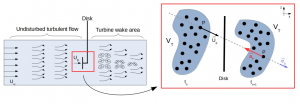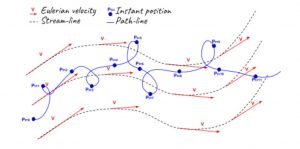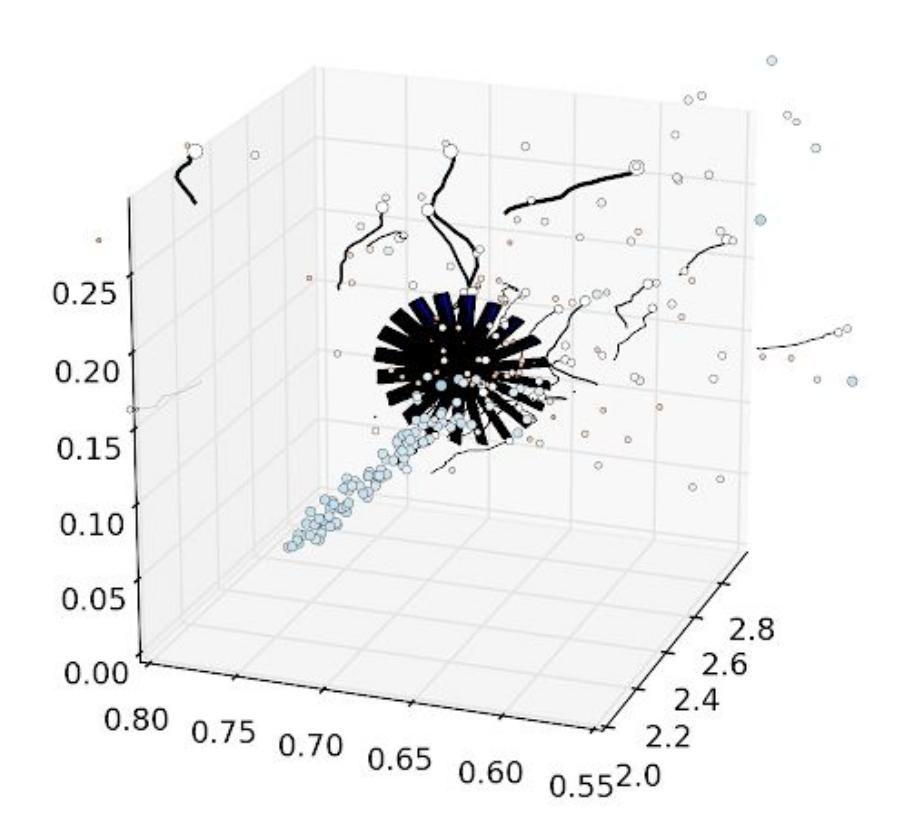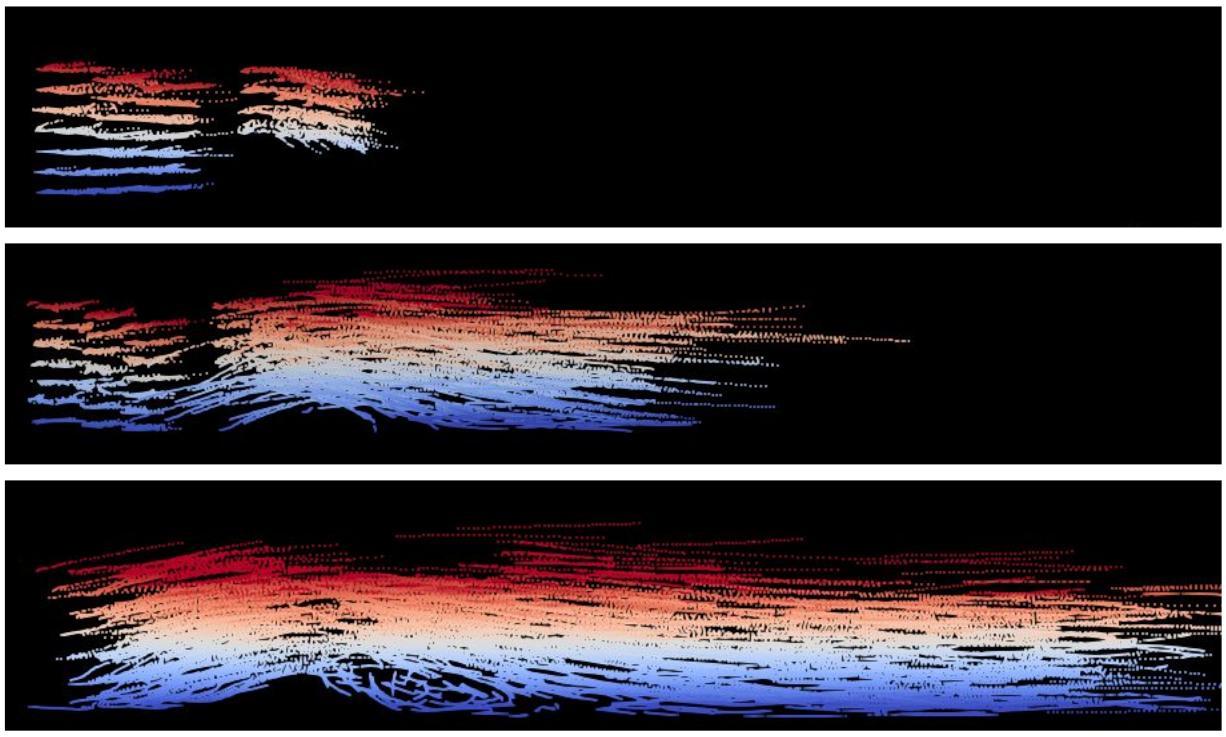# OceaPoS in SDM

The development of the SDM–OceaPoS model is based on an existing code that models air flows named
SDM–WindPos (validated on atmospheric flows, Bossy et al., 2016) that aims to reproduce basic turbulent flows generated in rivers and seas both upstream and downstream of an actuator disk device, by using a Lagrangian stochastic model. Therefore, both models share the following characteristics:

I- Stochastic: Uses a PDF description of flow model designed via the associated Fokker Planck Equation that allows local statistical analysis.

II- Particle algorithm:  The computational domain is divided in cells and each cell contains a number of particles defined by constant mass density constraint

III- Downscalable: Posibility refine the mesh and increase the bathymetry complexity in order to simulate reduced sections of the domain.

IV- Mirror wall-treatment: The method implemented consists of reflecting any particle that enters into a region close to the ground in order to reproduce the momentum exchange present in the interaction between fluid particles and arbitrary bathymetries.

# Models of hydro-kinetic turbines

• Non-Rotative actuator discsA new turbine model has been implemented based on the porous disc theory. This model can reproduce with quantitative concordance all the features measured for channel flows with actuator discs used in the recent experiments of Myers and Bahaj (2010, 2012).

The force exerted ona parcel of the disk is generally obtained  considering the disk volume. Alternative to this, SDM-OcePos proposes that the force is applied on a time depending volume of fluid  (Vt) that is determined by the amount of particles that cross the disk at a particular time step. The force acting on the group of particles depends on the thrust coefficient (Ct) of the hydro-kinetic turbine that is being represented by the actuator disk.

• Rotative actuator disks (under development)

In addition to the thrust, this model ntends to add a rotational force  on each particle that crosses the disk. This rotational force or moment depends on the torque coefficient (Cp) of the hydro-kinetic turbine represented by the actuator disk as well, turbine rotation  velocity and distance between the particle’s impact position on the disk to the rotations center.

# SDM-Particle tracking Visualization toolsStream-lines, tangent to the grid’s velocity vectors, and path-lines, defined by particle consecutive instant positions [3-c].

As an alternative to the wide range of classical numerical modeling, the numerical Stochastic Downscaling Method SDM-OceaPoS code presents an innovative and effective approach to simulate turbulent flows around marine current energy converters (MCEC), where the behavior of individual fluid particles is described as a stochastic process. However, this approach being relatively new, there is a lack of available tools, both in commercial and open source libraries, capable to exploit the results obtained from the Lagrangian nature simulation.(i) ROC-Meyers pre-visualization tool through python library “matplotlib” [3-c]. (click image for animation)

A visualization tool was created capable of recording the consecutive position  of a pre-selected set of fluid particles from output file of the SDM-OceaPos model in order to reproduce the particle’s trajectories.

As these trajectories withhold an accurate description of the physical processes involved in the flow, it is possible to assess how the particle’s behavior affected by the Lagrangian algorithm.

Once the particle trajectory files and list have been created, two type of output files are generated from the post-process python program: (i) python images and (ii) .VTK files.(ii) Almeida .vtk file visualization through paraview [3-c]. (click image for animation)

As it is intended to analyze the flow involving bathymetry profiles and solid 3-dimensional obstacles (such as porous disks or rotating mills, for instance), it is necessary to extract the data from the simulation of pre-selected particles at definite periods of time and in particular sections of the domain. Therefore, a set of new elements were introduced in the SDM source code in order to characterize particle pathline (see figure 1 ) . Additionally,
a new external module is developed to process the extracted data and reproduce the particle trajectories.